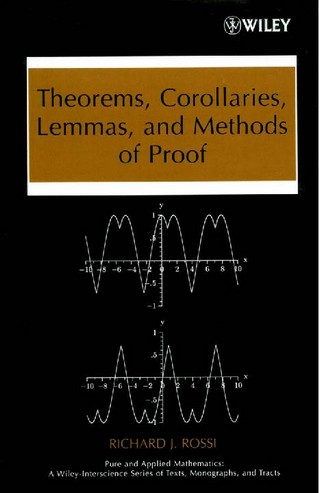### Book Toankho

Sách hay - Sách đẹp

# Theorems, Corollaries, Lemmas, and Methods of Proof (Pure and Applied Mathematics)A hands-on introduction to the tools needed for rigorous andtheoretical mathematical reasoningSuccessfully addressing the frustration many students experience asthey make the transition from computational mathematics to advancedcalculus and algebraic structures, Theorems, Corollaries, Lemmas,and Methods of Proof equips students with the tools needed tosucceed while providing a firm foundation in the axiomaticstructure of modern mathematics.
This essential book:
* Clearly explains the relationship between definitions,conjectures, theorems, corollaries, lemmas, and proofs
* Reinforces the foundations of calculus and algebra
* Explores how to use both a direct and indirect proof to prove atheorem
* Presents the basic properties of real numbers
* Discusses how to use mathematical induction to prove atheorem
* Identifies the different types of theorems
* Explains how to write a clear and understandable proof
* Covers the basic structure of modern mathematics and the keycomponents of modern mathematics
A complete chapter is dedicated to the different methods of proofsuch as forward direct proofs, proof by contrapositive, proof bycontradiction, mathematical induction, and existence proofs. Inaddition, the author has supplied many clear and detailedalgorithms that outline these proofs.
Theorems, Corollaries, Lemmas, and Methods of Proof uniquelyintroduces scratch work as an indispensable part of the proofprocess, encouraging students to use scratch work and creativethinking as the first steps in their attempt to prove a theorem.Once their scratch work successfully demonstrates the truth of thetheorem, the proof can be written in a clear and concise fashion.The basic structure of modern mathematics is discussed, and each ofthe key components of modern mathematics is defined. Numerousexercises are included in each chapter, covering a wide range oftopics with varied levels of difficulty.
Intended as a main text for mathematics courses such as Methods ofProof, Transitions to Advanced Mathematics, and Foundations ofMathematics, the book may also be used as a supplementary textbookin junior- and senior-level courses on advanced calculus, realanalysis, and modern algebra.